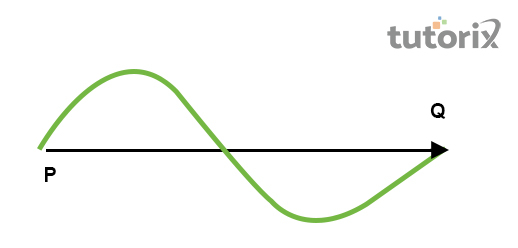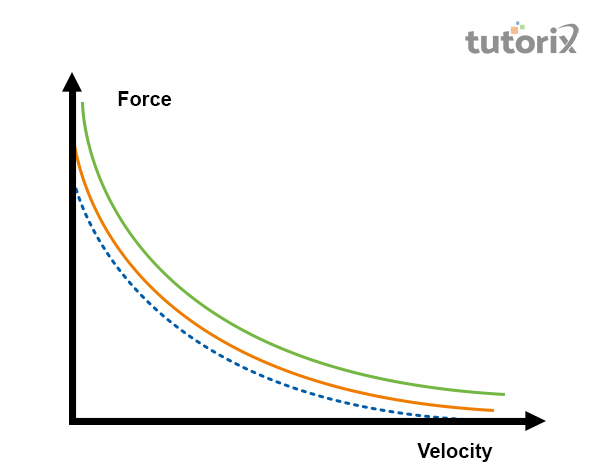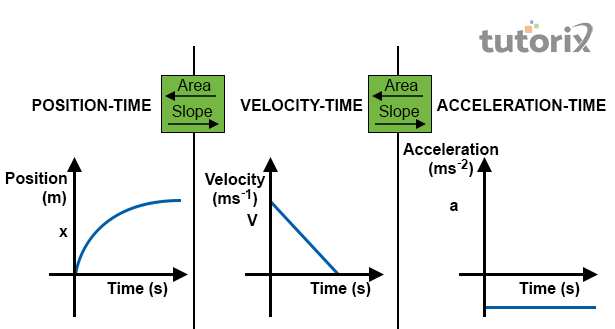# Difference Between Speed and Velocity

## Introduction

The similarity of speed and velocity of an object is the movement and the displacement from the previous position within a particular time. The SI unit of both particles is m/s which is a meter per second. Speed and velocity both are dependent on the distance covered, total time taken and direction of movement. The difference is also seen in the unit of measurement of these two aspects. The measuring unit of speed and velocity is meter/second.

## What is speed?Figure 1: Speed and velocity

Speed refers to the total distance covered by an object over a particular period. The lack of different directional components refers to the scalar quantity. Order to measure the average speed of an object relied on the distance travelled. It is also referred to as the rapidity of an object and the distance changing rate. Speed can be zero in any case and the speed of an object is never negative. The formula of the speed is Average speed = Total distance travelled / time taken (Xu et al. 2020).

For example, when a car is moving simultaneously side by side with a bicycle, and then the speed of the total distance covered is calculated by the vehicle within a specific time.

## What is velocity?Figure 2: Velocity

Velocity refers to the direction-aware of an object along with the rate of position changing. It belongs to the vector quantity having both magnitudes as well as directions. Velocity is measured by the difference in speed with the difference in time. It can be calculated with the formula Average velocity = Displacement/time taken. Velocity can be zero or negative as well as positive. For example, moving car returns to its primary position after covering a distance then the velocity of the car will be zero.

Velocity is all about the speed of an object that drives in the same direction (Xu et al. 2020). The formula for measuring velocity is $\mathrm{v\:=\:\Delta \:x\:/\Delta\:t\:}$ where v stands for the velocity, $\mathrm{\Delta \:x\:}$ refers to the displacement of the object and $\mathrm{\Delta \:t\:}$ denotes the arrival time.

### Different types of speeds

Speed can be classified into several categories like instantaneous, average, rational as well as tangential speed. The instantaneous speed is referred to as the instant speed. It can be measured by the formula $\mathrm{V=|v|\:=\:|dr/dt|}$.

The average speed is different from the previous and it refers to the total distance covered by an object in a specific time.

The rotational speed refers to the number of objects that turn in a specific unit of time.

The tangential speed refers to the speed of an object that moves along a circular path (Ivanov et al. 2019). The formula of this speed is $\mathrm{Tangential\:Speed\:=\:rotational\:speed\:*\:radial\:distance\:or\:v\:=\:r \omega\:}$ where v refers to the tangential speed, r stands for rotational speed and $\mathrm{\omega}$ refers to the radial distance. Other types of speed are uniform and non-uniform speed.

### Different types of velocity

Velocity discusses the object’s motion which helps in calculating the object’s movement based on the direction and speed. It is defined as a vector quantity which illustrates both direction and speed. The following are the types of velocity listed below−

• Constant velocity − The materials with constant velocity do not alter in direction or speed. The only materials that qualify as rotating at the continuous velocity are those that shift the straight line at the highest speed (Sauerheber & McCallum, 2020). The material in interstellar space outside a solar system is not under the impact of outside forces.

• Changing velocity − Transformation in the objects' velocity is calculated as acceleration. The materials with transforming direction and continuous speed are accelerating (Sciencing, 2022). Asteroids and comets within the solar system are the instances of objects with transforming velocity since the direction or speed is impacted by gravity.Figure 3: Motion graphs for velocity, acceleration and position

The above picture represents the story of the motion's object. The position, acceleration and velocity of the objects have been graphed over the interval of similar time, the shapes of every graph relate in a predictable and particular manner.

• Instant velocity − It is the procedure for demonstrating how rapidly the material is transforming its direction or speed at a particular point in time. It is comprehended by minimising the time utilised to calculate the acceleration to a few quantities that the object does not move during the time.

• Terminal velocity − It is basically used to discuss the object's movement falling freely through the air. The falling of objects to the ground in a vacuum may continuously accelerate till they point to the ground. The falling of objects through the air may stop moving because of the rising amount of resistance of air (Lanzilao & Meyers, 2022). A certain point at which resistance of air equals the velocity caused by gravity is defined as terminal velocity.

### Difference between Speed and Velocity

Speed Velocity
It is a Scalar quantity It is a vector quantity
It is described as a change of distance rate. It is illustrated as the change displacement rate.
It measures quantitatively how rapidly anything is shifting. It illustrates the movement direction of an object or body
No possibility of obtaining a negative value Possibility of getting Zero.
It is a prime indicator of object rapidity. It indicates position with object rapidity.

Table 1: Distinction between velocity and speed

## Conclusion

Velocity calculation is an extremely crucial utilisation in mechanical physics. It is often represented as vector quantity which is the speed at which an object travels in a specific direction. The scalar term defines the absolute magnitude of the value of velocity where the vector demonstrates motion speed. Speed is the distance covered by the object over a particular time irrespective of travelling distance. A directional component's lack means to be a scalar quantity. Calculating speed is easy which is the total distance travelled divided by the required total time to travel.

### FAQs

Q1. What is average velocity?

The location's change rate concerning time is known as velocity. It is also mentioned to be constant velocity in underlying the distinction from the average velocity. The formula is represented as $\mathrm{v = \frac{\Delta x}{\Delta t}}$.

Q2. What is the instance of speed and velocity?

Considering a dog runs at 10 km per hour which represents the speed. However, Consideration of running in some particular direction is velocity.

Q3. What is the SI unit of speed and velocity?

The SI unit for speed is known to be metre per second. The unit for velocity is similar to that of speed.

Q4. What is the main objective of the velocity-time graph?

The graph of Velocity-time is utilised for illustrating the continuous object’s acceleration. It is also called Speed time graphs.

Updated on: 13-Oct-2022

1K+ Views